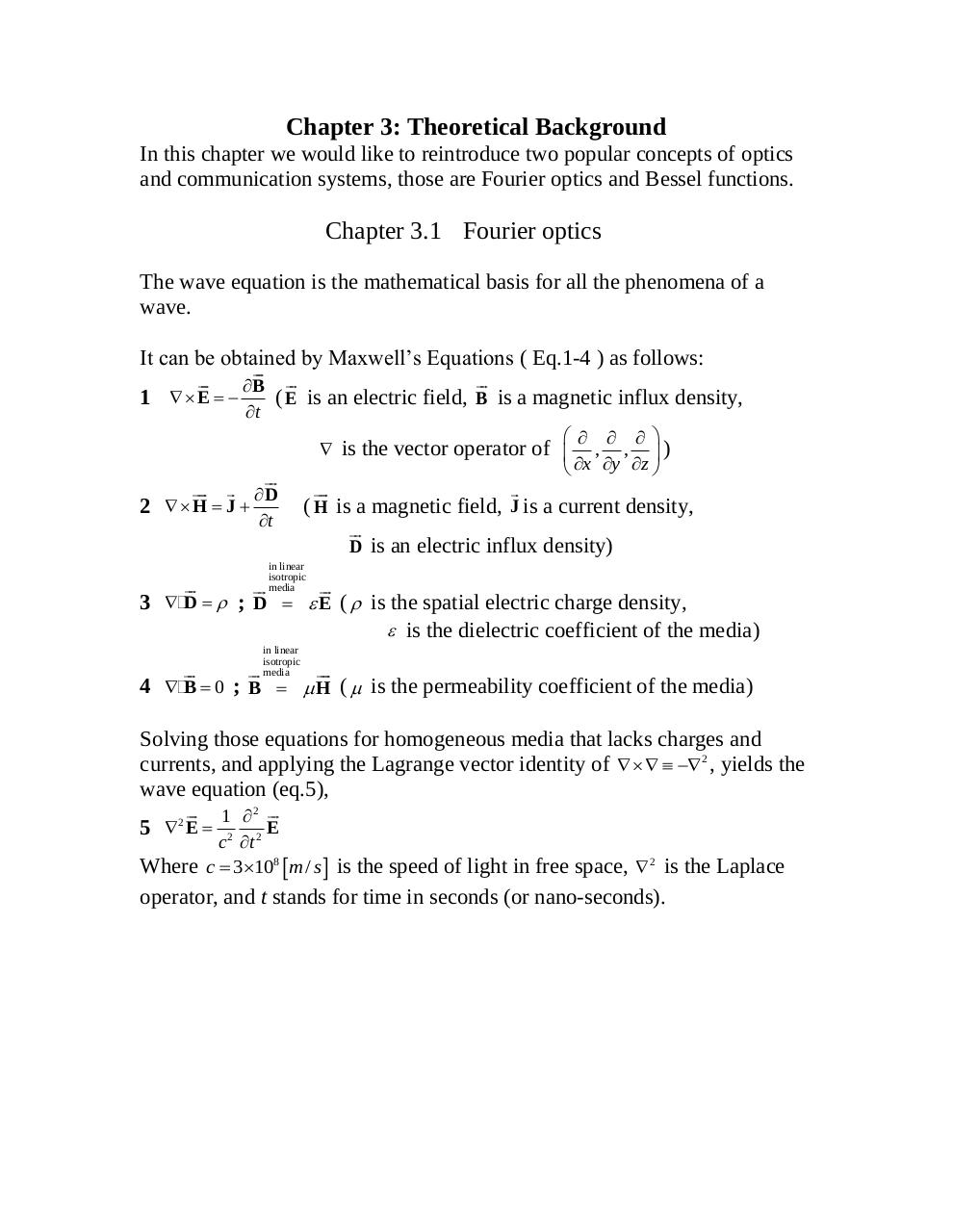# Project 14 2 1 839G1.22.pdfPage 1...3 4 56746

#### Text preview

Chapter 3: Theoretical Background
In this chapter we would like to reintroduce two popular concepts of optics
and communication systems, those are Fourier optics and Bessel functions.

Chapter 3.1 Fourier optics
The wave equation is the mathematical basis for all the phenomena of a
wave.
It can be obtained by Maxwell‟s Equations ( Eq.1-4 ) as follows:




 B 
1 E  
( E is an electric field, B is a magnetic influx density,
t
  
 is the vector operator of  , ,  )
 x y z 

   D


2 H  J 
( H is a magnetic field, J is a current density,
t

D is an electric influx density)




in linear
isotropic
media



3  D   ; D   E (  is the spatial electric charge density,
 is the dielectric coefficient of the media)
in linear
isotropic


 media 
4 B  0 ; B   H (  is the permeability coefficient of the media)

Solving those equations for homogeneous media that lacks charges and
currents, and applying the Lagrange vector identity of   2 , yields the
wave equation (eq.5),
 1  2 
5  E 2 2 E
c t
Where c  3108 m / s is the speed of light in free space,  2 is the Laplace
2

operator, and t stands for time in seconds (or nano-seconds).